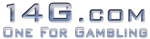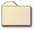SportsCasino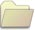LottoBingoPoker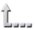Home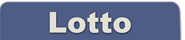Mathematics of Lotto

Mathematics of Lotto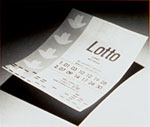Lotteries have the worst odds of any form of gambling, with a chance of approximately 1 in 14 million for the big win when playing most lottery jackpot games. However, they promise the greatest potential payoff to the winner with prizes regularly amounting to millions of dollars.Scratch-Off Tickets
In instant scratch-off games, the state takes about 50% of the action. 34% going to public education and the rest covering administrative and promotional costs. The remaining 50% is returned in prizes. Since the state keeps 50 cents of every dollar bet, the house edge is 50%, making the scratch-off game worse than any game in Nevada.

To find the probability of winning a particular prize, take the number of tickets for that particular prize and divide by the total number of tickets.

"Probability of Winning" = "No. of Winning Tickets" / "Total Amount of Tickets"

Example: Win and Spin.
Win and Spin was a typical California Lottery instant scratch-off game. There were 135 million tickets printed for Win and Spin, with 22,768,623 winners. This made the chance of winning equal 22,768,623/135,000,000, or about 1 in 6. Here are the Win and Spin payoffs, number of tickets of each type, and probabilities.

 Instant Prize (in \$) No. of Winners Probability or 1 10,800,000 0.083 1 in 12 2 8,100,000 0.062 1 in 16 5 3,240,000 0.025 1 in 40 10 540,000 0.004 1 in 241 50 54,000 4.153-4 1 in 2,407 100 27,000 2.076-4 1 in 4,815 500 6,073 4.672-5 1 in 21,406 1,000 1,350 1.038-5 1 in 96,296 10,000 150 1.154-6 1 in 866,667 Big Spin 50 3.846-7 1 in 2,600,000

To find your expected payoff, multiply each payoff by the number of winners, add, and divide the resulting sum by the total amount of tickets.

"Expected Payoff" = [Sum ("Payoff" x "No. of Winning Tickets)] / "Total Amount of Tickets"

where [Sum ("Payoff" x "No. of Winning Tickets)] simply is the total amount of prizes given away.

Example: Win and Spin.
[(1 x 10,800,000) + (2 x 8,100,000) + (5 x 3,240,000) + (10 x 540,000) + (50 x 54,000) + (100 x 27,000) + (500 x 6,073) + (1,000 x 1,350) + (10,000 x 150)] / 130,000,000
=
[10,800,000 + 16,200,000 + 16,200,000 + 5,400,000 + 2,700,000 + 2,700,000 + 3,036,500 + 1,350,000 + 1,500,000] / 130,000,000
=
59,886,500 / 130,000,000
=
0.46066538 or ca. 0.46

The result is an expected payoff of about +\$.46. Now you have to subtract the prize for the ticket. Subtracting let's say one dollar you paid for the ticket leaves you with expected winnings = -\$.54.Lotto
In order to develop a formula for computing lotto probabilities, we must get familiar with the world of combinatorics (counting).

Example: Lotto: Pick 6 out of 49 numbers.

The question is: What is the chance that 6 of your 6 picks are amongst the 6 winning numbers?
The next question is: What is the chance that 5 of your 6 picks are amongst the 6 winning numbers?
And so on.

To start with we first need to know in how many ways 49 numbers can be drawn. The first ball could be any of 49 available balls. The second ball could be any of 48 available balls (because one is drawn already). The third ball could be any of the 47 remaining balls. The next ball could be any of 46 remaining balls. And so on. All the way down to the last of the 49 balls. Thus there are 49 x 48 x 47x 46 x 45 x 44 x 43 x 42 x 41 x ... x 1 = 6.0828262 ways in which 49 balls can be drawn. Mathematically you say there are "49 factorial" ways and write "49!".

Now we need to know in how many ways you can draw 6 out of 49 numbers. We don't ask for the chance to draw 6 winning numbers yet. Could be any number. Well, there are n! / k! (n_k)! ways to select k numbers from n numbers. This formula is read as "n choose k" and for convenience denoted by (nk). In our example there are (496) = 49! / 6! (49-6)! = 13,983,816 or nearly 14 million ways to select 6 out of 49 numbers.
So the chance of winning the jackpot is 1 in 14 million. To put these odds in perspective, if you buy 50 lotto tickets a week, you'll win the jackpot about once every 5,000 years. Or suppose you are in a football stadium filled with 70,000 people, and there are 200 such stadiums. Select one person at random from these 200 stadiums. Your chance of being selected is the same as your chance of winning the Lotto jackpot.

Now we want to know what the probability is that only 1of 6 select numbers is a winning number:

a) There are (496) = 13,983,816 ways to select 6 out of 49 numbers.

b) There are 6 winning numbers. So there are (61) = 6! / 1! (6-1)! = 6 ways to select 1 out of 6 winning numbers.

c) There are 49 - 6 = 49! / 6! (49-6)! = 43 numbers that don't win. So there are (435) = 43! / 5! (43-5)! = 962,596 ways to select 5 out of 43 numbers that don't win.

d) This gives a total of (61) x (435) = 5,775,588 ways to select 6 out of 49 numbers, with one that is a winning number.

To get the probability of having one number correct you just need to divide "the number of ways to select 6 out of 49 numbers, with one that is a winning number" by "the total amount of ways to select 6 out of 49 numbers". Thus,

 P(0 winning numbers) = (60) x (436) / (496) = 6,096,454 / 13,983,816 = 0.435965 or 1 in 2 P(1 winning number) = (61) x (435) / (496) = 5,775,588 / 13,983,816 = 0.413019 or 1 in 2 P(2 winning numbers) = (62) x (434) / (496) = 1,851,150 / 13,983,816 = 0.132378 or 1 in 8 P(3 winning numbers) = (63) x (433) / (496) = 246,820 / 13,983,816 = 0.0176504 or 1 in 57 P(4 winning numbers) = (64) x (432) / (496) = 13,545 / 13,983,816 = 0.00096819 or 1 in 1,032 P(5 winning numbers) = (65) x (431) / (496) = 258 / 13,983,816 = 1.844e-05 or 1 in 54,201 P(6 winning numbers) = (66) x (430) / (496) = 1 / 13,983,816 = 7e-08 or 1 in 13,983,816

To compute the expected payoff for a lottery ticket, multiply the payoffs for each number_match by their probabilities to occur and add them all together. Subtract from this amount the price for the ticket.
Since the payoffs are depending on a) how much money the lottery pot contains, and b) how many people have won, and splitting prize money with the winners means that there are no fixed payoff odds, it is not possible to calculate the expected payoff for each lottery ticket you buy.

As with instant scratch-off games, 50% of the money bet on Lotto is kept by the state and 50% is returned in prizes. In a sense, the house edge is 50%.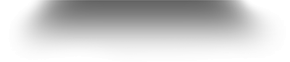Home